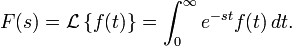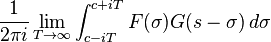# Laplace Transform of f(t) x cos(t)

alanifamily

## Homework Statement

Find the Laplace transform of f(t) x cos(t) (multiplication) if the Laplace transform of f(t) is F(s).

## Homework Equations## The Attempt at a Solution

I'm pretty sure I am supposed to use the second equation, however I do not understand how to use it. If you could give me an example on how to find the Laplace Tranform of f(t) x g(t) that would be very helpful.

Thank you